American Journal of Civil Engineering
Volume 3, Issue 2-2, March 2015, Pages: 57-59

Comparison Between Experimental Modelling and Analytical Solution of Dam Break

Amir Heshmati-Far1, *, Amirhossein Roohezamin2, Amir Mahmoudi Anzabi3

Department of Civil Engineering, Sharif University of Technology, Tehran, Iran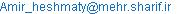(A. Heshmati-Far)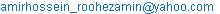(A. Roohezamin)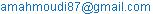(A. M. Anzabi)

Amir Heshmati-Far, Amirhossein Roohezamin, Amir Mahmoudi Anzabi. Equation Chapter 1 Section 1 Comparison Between Experimental Modelling and Analytical Solution of Dam Break. American Journal of Civil Engineering. Special Issue: Research and Practices of Civil Engineering in Developing Countries. Vol. 3, No. 2-2, 2015, pp. 57-59. doi: 10.11648/j.ajce.s.2015030202.21

Abstract: The dam break is a very important phenomenon that occurs in the dams as one of the most important hydraulic structures. This study has tried to model this phenomenon experimentally and the results of the experiments are compared with the response of the analytical solution of characteristic method. The experiments are done in both dry and wet downstream. Finally, sources of error which cause a slight difference between the experimental and theoretical results are discussed.

Keywords: Dam Break, Characteristic Method, Experimental Modelling

Contents

1. Introduction

Dams are one of the most important structures made by men. Despite of numerous advantages of dams, because of the possibility of dam-break, they are potentially dangerous and their failure causes a lot of casualty. Therefore, beside the precise and safe design, the dam-break should be modeled and be studied carefully.

The investigations done by Ritter  about the dam-break is one of the first studies conducted in this field. Based on the Saint-Venant equations some relations are proposed in this study, but defying the friction of riverbed and hydraulic resistance of turbulent stream causes the proposed relations lacks preciseness and validation, and if the terms related to those factors are added to the Saint-Venant equations, the final relations cannot be solved due to complexity.

A simple and new solution for the dam-break problem is proposed in Chanson’s studies . In his study, based on the method of characteristic, for a semi infinitive reservoir, a new solution of the "laminar wave" due to the dam-break is suggested. The study is conducted for a dry downstream riverbed, and the riverbed is considered both horizontal and sloped and the fluid is assumed ideal. Based on these assumptions, the position and the velocity of the frontal wave and the instantaneous free surface profile are presented. Compared to the experimental results, the proposed solution is precise and acceptable.

In some studies such as Schoklitseh  and Estrade and Martino-lagarde , the turbulent wave due to the dam-break is experimentally investigated. This wave is also studied analytically by Witham  and Hunt . In the studies of Hunt , Aguirre-Pe et al  and Debiane  the wave due to dam-break is considered as a laminar wave. In the Hunt’s study , an analytical solution based on the kinematic wave and for a sloped riverbed is proposed for the dam-break problem. The dam-break problem in a research conducted by Aguirre-Pe et al  is investigated both numerically and experimentally for a sloped riverbed. In Debiane’s  research, riverbed is considered horizontal and sloped.

Despite of the studies done in this field, there are still some deficiencies in the basic theory and physical investigation. For instance, the information about the "surge" due to the dam-break is little. The new predictions of the dam-break is highly dependent on the numerical predictions which are valid based on some limited data.

In this research, the wave due to the dam-break is investigated by conducting an experiment and simulating the dam-break phenomenon. The results are compared with the theoretical analysis of the dam-break, which is based on the Saint-Venant’s equations. Accordingly, in a flume with specified dimension, a dam and its reservoir are simulated. In this flume, a gate plays the role of the dam and the dam-break is simulated by removal of the gate. By processing the images, captured the wave formed in the flume, the resultant free surface profile can be obtained and compared with the profile gained by Saint-Venant’s equations. Also the velocity of the wave front and the volume of the displaced water gained experimentally and theoretically and can be compared with each other.

2. The Method of Characteristic

Due to the nonlinearity and complexity of the existing equations, the Saint-Venant’s equations cannot be solved explicitly and the depth and velocity of the flow cannot be computed. By using the semi graphical "method of characteristic" both the explicit and the numerical solution are obtained. By combination of the equations associated with Saint-Venant’s equations, the new equations are written as: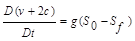(1)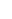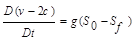(2)

Where S0 and Sf are bed and energy line slope, respectively, g is the acceleration of gravity and c and v are the wave and the fluid velocity, respectively. The equations (1) and (2) illustrate the forward and backward characteristic, respectively. If S0 =Sf =0, then the equations (1) and (2) turn into: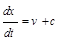(3)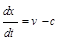(4)

The studies are conducted for both wet and dry downstream riverbed. By using the method of characteristic, the velocity of the wave front in the dry downstream riverbed case is calculated as: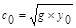(5)

Where y0 is the height of the reservoir before dam breaks and c0 is the velocity of the wave formed by the backwater flow toward the dam upstream. Based on the Saint-Venant’s equations and the method of characteristic, the depth as a function of time and position is calculated as: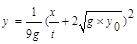(6)

Where x is the distance from the gate and t is the time duration after removal of the gate (dam-break). Accordingly, the depth (y) and the flow velocity (v) exactly at the gate position (x=0) are calculated as: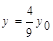(7)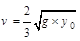(8)

The above equations represent the dry downstream riverbed case. For the wet case, the governing equations are: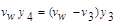(9)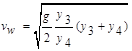(10)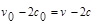(11)

The y4 is the depth of the downstream water before the dam-break, vw is the velocity of the wave front (surge) and y3 and v3 are the depth and flow velocity in the negative wave region, respectively. The v0 and c0 are the initial conditions.

3. Experimental Modeling

For modelling the dam-break and the associated wave, a Plexiglas tank of cross section 30 x 15 cm and length of 70 cm is used. A gate installed in the middle of the channel works as a dam and by removing it abruptly, the dam-break is simulated. It is very important the gate be raised "abruptly" because slow removal of the gate leads to ill modeling of the dam-break especially in the case of the wet downstream riverbed. Before conducting the experiment, all of the turbulence should be vanished because all the relations are based on the zero initial velocity. For a better vision of the flow in the channel, a colored material like Permanganate should be applied. All of the dam-break procedures should be filmed and turned into the images by "ImageGrab" software. By using "Plot Digitizer", the free surface profile of the flow in each moment is obtained.

4. Results

Experiments for the dry downstream riverbed case has been performed and summarized in the table 1. The profile of characteristic of dry downstream is shown in Figure 1.The slope of the theoretical profile is the speed of frontal wave that it shown by vc, also this speed is calculated with experimental data and equation (5) that is called ve.

Table 1. Specifications of experiments for dry downstream.

 Experiment No. Depth of water (y0) Vc (m/sec) Ve (m/sec) Experimental 1 11.5 cm 2.12 1.45 Experimental 2 12.5 cm 2.22 1.56 Experimental 3 13.5 cm 2.30 1.62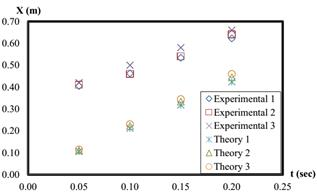Figure 1. The characteristics of dry downstream.

Also we done three experiments for the wet downstream that its results is summarized in table 2. The profile of characteristics of the wet downstream is shown in fig. 2. In this case, the speed of frontal wave isn’t equal with slope of profile of characteristic in the state of theory. We used the equation (10) to calculate frontal wave speed.

Table 2. Specifications of experiments for wet downstream.

 Experiment No. y3 y4 Vc (m/sec) Ve (m/sec) Exp1 15.5 cm 4 cm 0.9190 1.54 Exp2 16.5 cm 4 cm 0.9400 1.62 Exp3 17.5 cm 4 cm 0.955 1.77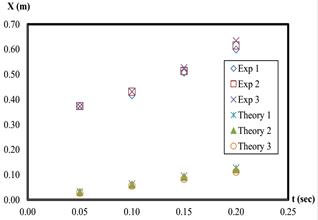Figure 2. The characteristics of wet downstream.

5. Discussion and Conclusion

In characteristics method, the origin of place is the position of gate. Now, if the origin of the theoretical characteristic profile is transported to x = 0.35 m then there is a good likeness between theoretical and experimental results. Also, if we fitted a line on the experimental data, it cut the position axis at the around x = 0.35 m. There are many sources of error which cause a slight difference between experimental results and theoretical results. Some of them are:

a)  The most important factor of creation of error is the effect of friction of the channel bottom and the walls. This parameter affect on the velocity that we ignored it.

b)  There is a bulge at the gate place in the experimental setup. This bulge creates a flow like flow crossing over the spillway and causes small error.

c)  The vertical acceleration of water during the raise of the gate.

d)  Speed of the gate when it is raised.

References

1. A. Ritter, "The propagation of water waves," Ver Deutsch ingenieur zeitschr, 36, Pt. 3, No. 33, 947–954, 1982.
2. H. Chanson, "Application of saint-venant Equations and method of Characteristics to the dam break wave problem," Report No. CH55/05, Dept. of civil Engineering, the university of Queensland, Brisbane, Australia, Mey 2005.
3. A.Schoklitseh, "UberDambruchwellen." Situngberichten der koniglicheAkadewis der wissenschaften, viena, vol. 126, part IIa, pp- 1489-1514, 1917.
4. J. Estrade, A. and Martino-lagarde, "Ecoulement consecutive a la suppression d’um Barrage dans un canal Horiontal de section Rectangularie." Comptes.Rendus de l’Academie des sciences de Paris, vol. 259, 21 December, Group 2, pp. 4502-4505, 1964.
5. G.H. Witham, "The Effects of Hydraulic Dam-Break Problem."Proc.Roy. Soc. of Landon, Ser.A, vol. 227, pp.399-407, 1995.
6. B. Hunt, "Asymptotic solution for Dam-Break Problem."JI of Hyd. Div., Proceedings, ASCE, vol. 108, No.HYI, pp.115-126, 1982.
7. B. Hunt, "Newtonian Fluid Mechanics Treatment of Debris Flows and Avalanches."JI of Hyd. Engrg., ASCE, Vol. 120, No.12, pp.1350-1363, 1994.
8. J. Aguirre-Pe, F.P. Placheo, S. Quisca, "Test and Numerical One-Dimensional Modeling of a High-Viscosity Fluid Dam-Break Wave."JI pf Hyd. Res., IAHR, vol. 33, No.1, pp. 17-26, 1995.
9. K. Debiane, "Hydraulic des EcoulementsLaminarious a surface Libre dams un canal pour des Milieuxvisqueuxouviscoplastiques." Ph.D thesis, university of Grenoble I, Pheology Laboratory INPG-UJF-CNRS, Frances, 2000.

 Contents 1. 2. 3. 4. 5.
Article ToolsAbstractPDF(384K)